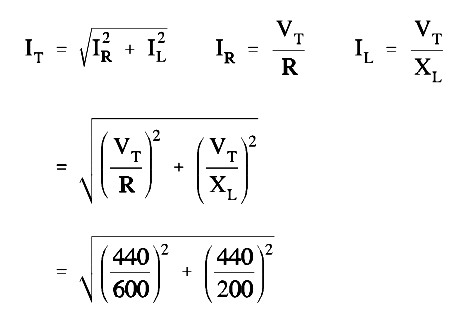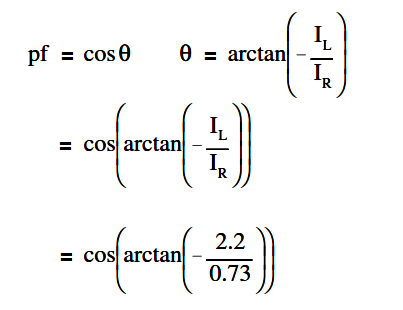# Calculate Power in Parallel RL Circuit

A 600 Ω resistor and 200 Ω XL are in parallel with a 440V source, as shown in Figure.Figure : Parallel R-L Circuit

Find:

1. Current, IT
2. Power Factor, pf
3. True Power, P
4. Reactive Power, Q
5. Apparent Power, S

Solution:

##### 1.Current, ITIT  = 2.3 amps

##### 2. Calculate Power factor (pf)p.f. = cos (- 71.5º)

p.f. = 0.32

##### 3. Calculate True Power, P

P = EI cos θ

P = (440)(2.3)(0.32)

P = 323.84 watts

##### 4. Calculate Reactive Power, Q

Q = EI sin θ

Q = (440)(2.3)(0.948)

Q = 959.4 VAR

S = EI

S = (440)(2.3)

S = 1012 VA